# Advanced Circuit Analysis and Exploration with Circuit Parameters in NI Multisim

Publish Date: Nov 22, 2017 | 3 Ratings | 4.00 out of 5 | Print | Submit your review

## Overview

NI Multisim allows engineers to optimize the performance of electronic circuits earlier in the design flow with simulation to ensure that circuits meet specifications with fewer prototype iterations. When designing analog and mixed-mode circuits, Multisim enables accurate and rapidly analysis of electronics behavior critical to performance. Multisim comes with a number of advanced analysis capabilities that allow it to be a premier research and analysis tools. Introduced in Multisim 13.0 are “circuit parameters” which adds sophistication to any simulation approach by allowing an engineer to easily explore different scenarios for circuit structures and component values, as well as perform advanced analyses such as temperature sweep, parametric sweep, and Monte Carlo analysis to validate design corner cases.

### 1. What Are Circuit Parameters?

Circuit parameters allow users to define parameters or variables that are assigned to values within a circuit topology. These circuit parameters can then be easily viewed, changed or swept to impact device values or can be setup to quickly calculate critical equations associated with a particular circuit. Because of the way that circuit parameters are setup device values can be dynamically evaluated during simulation (for example creating dependencies between frequency, RLC values etc…)

In this document we will be able to view how circuit parameters are set, how they are used and how they are an exceptionally power simulation tool to improve design performance.

In the schematic of Figure 1 we can see how circuit parameters are setup. You will notice that the passives R1, R2, C1 and C2 all do not have a value associated in them in the diagram. They are instead represented by alpha-numerics. In the corresponding circuit parameters dialog box you will see the “parameter name” of R1, R2, C1 and C2 – this is where the values are set. As you can see all of these circuit parameters have values based upon math expressions (for example being a function of frequency or the quality factor)

By placing circuit parameter values in this manner an engineer can quickly change a value, create a new expression to define the value or can dynamically change the value based upon various elements of a circuit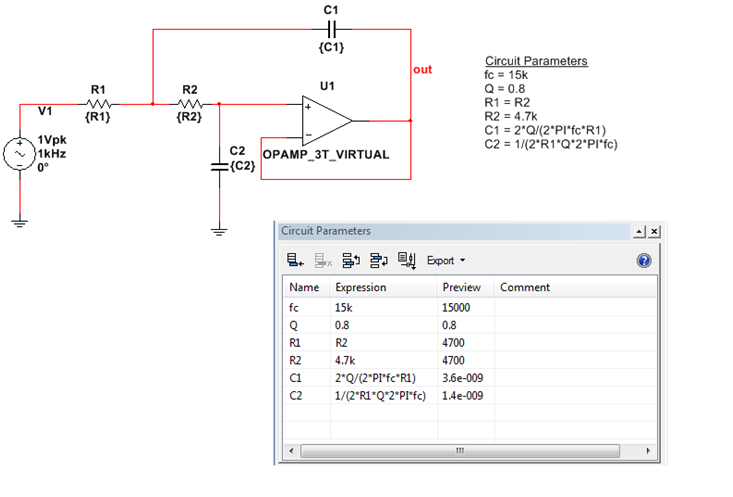Figure 1. Example circuit with defined circuit parameters

### 2. Example 1: How to Define Circuit Parameters for a Simple RLC Filter

To demonstrate how to properly setup circuit parameters we are going to consider the simple RLC circuit shown in Figure 2 as an example.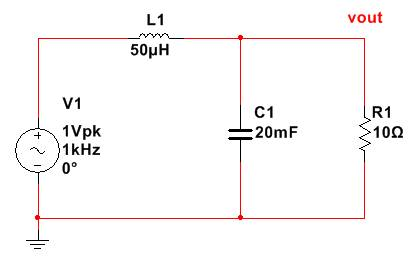Figure 2. Example RLC circuit used to demonstrate how to setup circuit parameters.

We are going to be investigating the effect of two important filter design variables:

• Cut-Off Frequency – frequency at which the amplitude is reduced by a 3dB corner.
• Quality Factor – Dimensionless parameter to describes the degree of damping.

Here we are going to set the value for the inductor, cut-off frequency and the quality factor and dynamically determine the required resistor and capacitor component values.

1. Open the circuit parameters panel through View » Circuit Parameters. This gives us a table through which we can configure our schematic level parameters.

2. Define the following parameters within the table:

 Parameter Name Expression Comment Fc 10k Cut-off Frequency L 10u Inductor Value Q 0.8 Quality Factor

These parameters are going to remain static. The resistor and the capacitor values are going to be determined dynamically based upon these values.

3. In order to determine the resistor and capacitor values we need to enter the corresponding expressions based on analog electronics fundamentals. Enter the following additional circuit parameter values:

 Parameter Name Expression Comment C 1/(2*PI*Fc)^2/L Capacitor Value R Q/sqrt(C/L) Resistor Value

Upon completion of the above expressions you will notice that Multisim calculates a preview of the values that will be used for R and C as in the image below: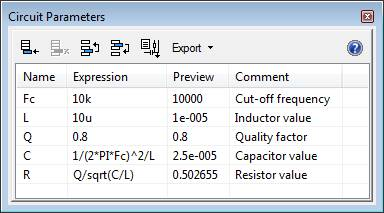Figure 3. Circuit Parameters tab in Multisim

4. Whilst we have defined our circuit parameters we still need to assign these to our components within the schematic. To do this, double click on the resistor in the schematic and set its value to be the corresponding circuit parameter name (b in this case) as in the image below. Repeat the process for the inductor and capacitor (with the corresponding L and C circuit parameters).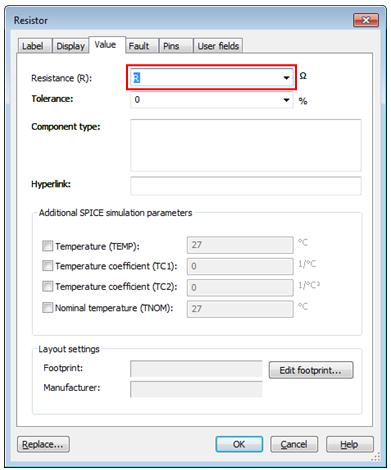Figure 4. Setting component value as a circuit parameter

Once assigned Multisim will use the circuit parameter value for the assigned components when simulating or running analysis models on the schematic.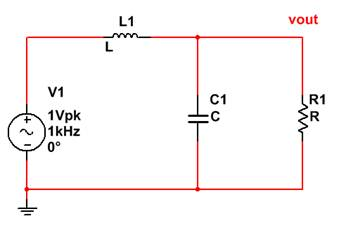Figure 5. Schematic components in Multisim with defined circuit parameters as values

5. One very useful aspect of circuit parameters is that we can change the value of one component and immediately see the affect this will have on other components. Try changing the value of the cut-off frequency (Fc) from 15k to 10k and view the effect this will have on the preview of the resistor and capacitor values (R and C). This allows designers to see the immediate effect of different design requirements on component values. Return the Fc value to 15k.

6. Now we will run an AC analysis to check if the cut off frequency is that which we specified. Select Simulate » Analyses » AC Analysis

7. Keep the defaults for the Frequency Parameters and click on the Output tab and select V(vout) from the Variables in circuit pane. Click add to use this for the analysis as shown below.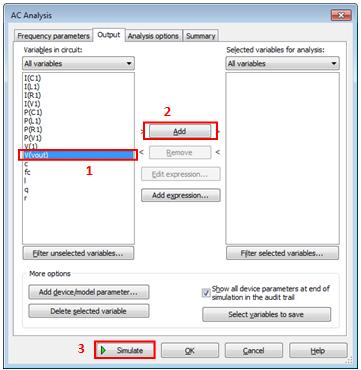figure 6. Running the AC analysis

8. Click Simulate to start the simulation.

9. This will produce a magnitude and phase graph as below, from which we can see the cut-off frequency is at 15kHz.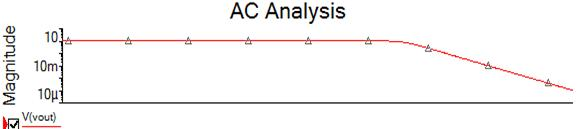Figure 7. AC analysis simulation result

9. We can also use circuit parameters to explore how key variables affect the response of a circuit. In this case we will explore the affect the quality factor Q has on the response. We can do this using the Parameter Sweep analysis.

10. Open the Parameter Sweep Analysis dialog box: Simulate» Analyses » Parameter Sweep.

11 .Configure the parameter sweep as displayed in the figure below. This is setup to sweep through a range of values to identify the affect the quality factor has on the AC response of the circuit.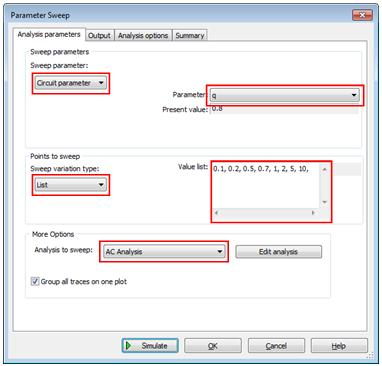Figure 8. Setting up a parameter sweep to run over a circuit parameter

12. Click on the output tab and select V(vout) to be the parameter for analysis as you did for the AC analysis previously. Click simulate to run the analysis.

13. The results are displayed on a graph allowing us to clearly see the response for various Quality Factor values.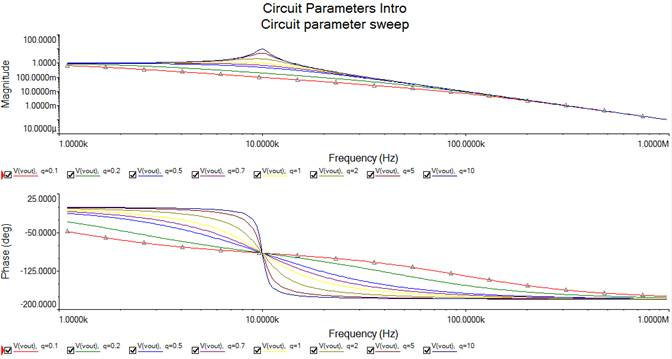Figure 9. Simulation result of the parameter sweep showing the effect of varying the filter quality factor on the AC response

### 3. Example 2: Advantages of Circuit Parameters for a High-Order Filter Simulation

In this example a high-order passive low-pass filter circuit is used to demonstrate the circuits parameters functionality. The circuit parameters allowed the accurate completion of the following tasks:

• Defining high level circuit parameters such as frequency
• Using circuit parameters in mathematical expressions to define new parameters
• The actual  of expression is shown in the Preview column which bears resemblance to a mathematical scripting tool. This column allows you to gain confidence in your equations; learn about how mathematical functions and operators in Multisim behave instantly. (You can see the table listing all the supported functions/operators in the help menu)
• Assigning the same circuit parameters to one or many components components
• Running a Parameter Sweep analysis on a circuit parameter, this effectively allows to sweep almost ANY numeric component/model parameter in the circuit
• The same way MonteCarlo and Worst Case analyses could be run

### Simulation Steps

1. Drag and drop the circuit snippet (PNG image) below to load the circuit in your Multisim environment
2. Notice how all the RLC components in the circuit are defined as generic parameters that could be easily swept
3. The advantage of this is saved time when trying different values of R, L, or C because now you only need to change the value of the global parameter instead of changing the value of every component
4. Go to Simulate>Analyses>Transient analysis and run the simulation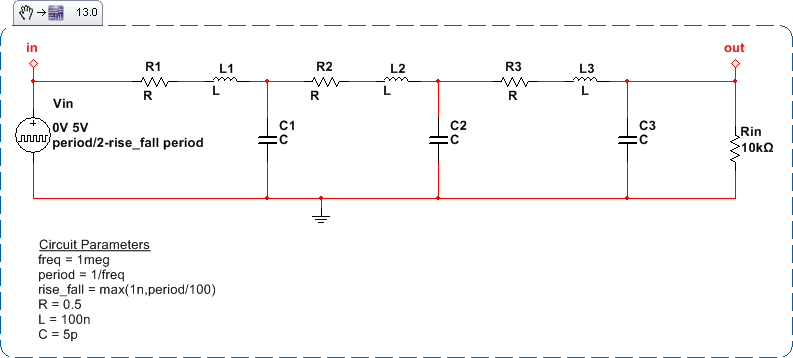Figure 10. Snippet of a multi-stage filter circuit where component values are defined using circuit parameters

4. Looking at the output voltage. The result is the filter response using a single value of circuit parameters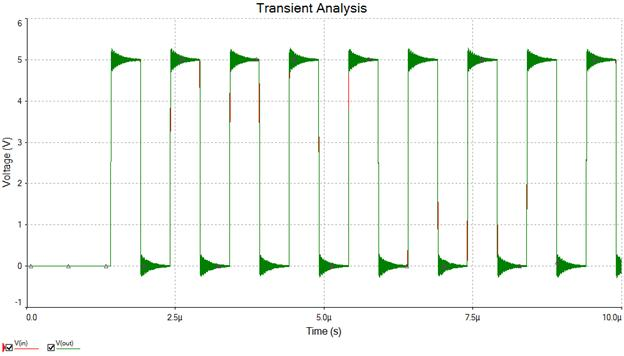Figure 11. Transient time response

6. Now setup a parameter sweep by going to Simulate>Analyses>Parameter Sweep and run the simulation again

7. The result shows how different parameter values affect the transient response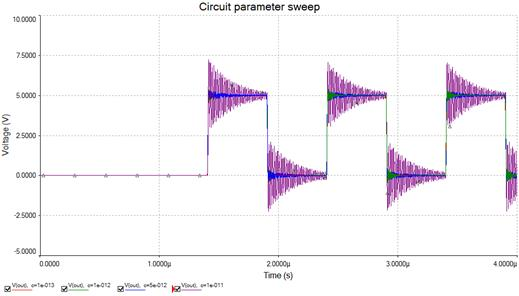Figure 12. Parameter sweep results iterating over the value of a circuit parameter

Multiple corner cases of this design topology could be evaluated using this approach.

### 4. Example 3: Evaluating Design Case Structures with Circuit Parameters

This example demonstrates a powerful way of evaluating different design cases and tuning multiple parameters of the circuit at the same time. The goal is to figure what component values will help meeting the design specifications.

The circuit is a Sallen-Key Low pass filter. The user can define high-level parameters for cut-off frequency and quality factor Fc and Q, which set the RC values. The table() function is used to perform a case-based study, where multiple parameters are adjusted to specific values in every case. The table() functions is just a piece-wise-linear look-up function.

The first parameter is the independent variable (Case), and the rest are pairs for the look-up, with linear interpolation done in between. For example if you set Case to 1,2,or 3 you will get the values of 15k,25k,and 40k respectively for Fc and 0.7,0.8,0.9 for Q. You will also see the Preview column changing accordingly in the Circuit Parameter tabs.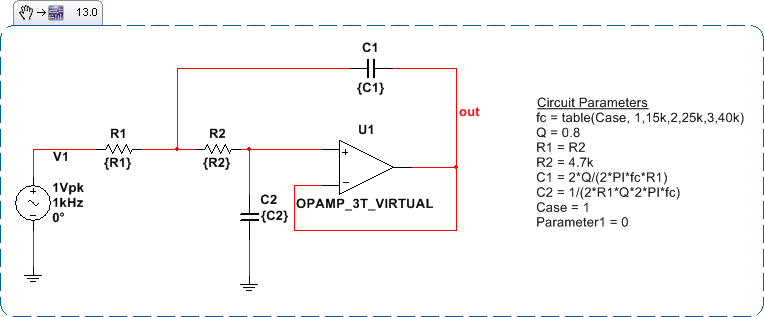Figure 13. Op-amp circuit with component values defined using a case structure for the circuit parameters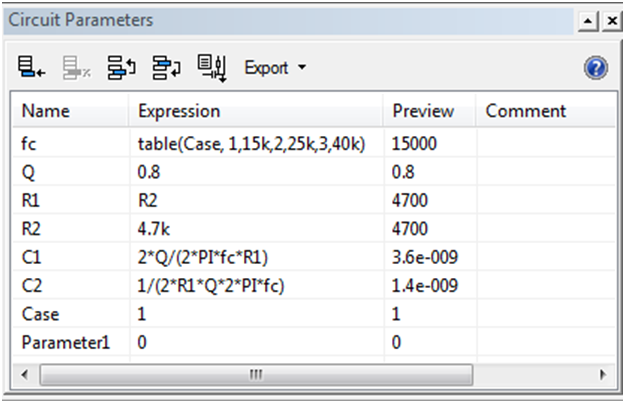Figure 14. Preview of the circuit parameters tab

### Simulation Steps

1. Drag and drop the snippet image above to Multisim to load the circuit
2. Go to Simulate>Analyses>Parameter Sweep
3. Run the simulation which will sweep the parameter Case and display different results of the filter response for each case with a different cutoff frequency and quality factor (fc and Q)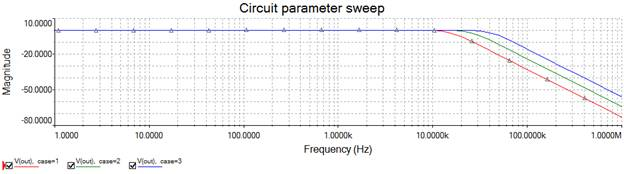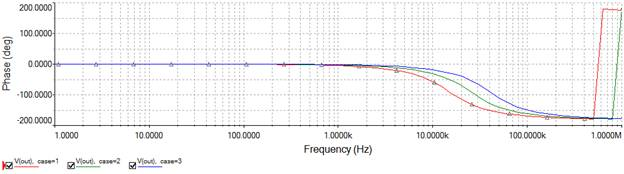Figure 15. Simulation results of sweep analysis over different cases of component values

### 5. Conclusion

Circuit parameters is a powerful tool to accurately model multiple values of components in a circuit before accurately simulating it. It provide schematic level variables that the user can assign to components, calculate key variables and use in analysis models. Together these allow designers to gain a deeper understanding of the circuit behavior and experiment with schematics to understand the full characterization of their designs.

### Ratings

Rate this document